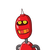# 11. The digits of a two-digit number differ by 3. If digits are interchanged and theresulting number is added to the origina

11. The digits of a two-digit number differ by 3. If digits are interchanged and the
resulting number is added to the original number, we get 121. Find the original
number.​

1.Let’s say, that the one’s place digit be y and ten’s place digit be x respectively.

• Original number = (10x + y).

⠀⠀⠀⠀⠀━━━━━━━━━━━━━━━━━━━⠀⠀⠀⠀

Given that,

• As per given condition, the digits of a two – digit number is differ by 3.

Then,

➟ x – y = 3

x = y + 3⠀⠀⠀⠀⠀⠀⠀⠀—eq. ( I )

⠀⠀⠀

$$\underline{\bigstar\:\boldsymbol{According~to~ the~Question :}}$$

⠀⠀⠀

• If digits are interchanged and the resulting number is added to the original number, we get 121.

⠀⠀⠀

Therefore,

⠀⠀⠀

$$\dashrightarrow\sf 10x + y + 10y + x = 121 \\\\\\\dashrightarrow\sf 11x + 11y = 121 \\\\\\\dashrightarrow\sf x + y = 11\\\\\\\dashrightarrow\sf y + 3 + y = 11\qquad\quad\bigg\lgroup\sf From\;eq^{n}\;1\bigg\rgroup\\\\\\\dashrightarrow\sf 2y + 3 = 11\\\\\\\dashrightarrow\sf 2y = 11 – 3 \\\\\\\dashrightarrow\sf 2y = 8\\\\\\\dashrightarrow\sf y = \cancel\dfrac{8}{2}\\\\\\\dashrightarrow\underline{\boxed{\frak{\pink{\pmb{\purple{y = 4}}}}}}\;\bigstar$$

⠀⠀⠀

⠀⠀⠀$$\underline{\bf{\dag} \:\mathfrak{Putting\; value \;of\; y \;in\;eq^{n}\;(1)\: :}}$$⠀⠀⠀⠀

⠀⠀⠀

$$\dashrightarrow\sf x = y + 3\\\\\\\dashrightarrow\sf x = 4 + 3\\\\\\\dashrightarrow\boxed{\frak{\pink{\pmb{\purple{x = 7}}}}}\;\bigstar$$

ORIGINAL NO. = (10x + y)

⠀⠀⠀

⇥ No. = 10x + y

⇥ No. = 10(7) + 4

⇥ No. = 70 + 4

No. = 74

⠀⠀⠀

∴ Hence, the required two – digit no. is 74.

$$\rule{250px}{.3ex}$$

V E R I F I C A T I O N :

• As it is given that, the digits of a two– digit number is differ by 3.

Therefore,

$$:\implies\sf x – y = 3 \\\\\\:\implies\sf 7 – 4 = 3 \\\\\\:\implies\underline{\boxed{\frak{ 3 = 3}}}$$

⠀⠀⠀

$$\qquad\quad\therefore{\pink{\underline{\textsf{\textbf{Hence, Verified!}}}}}$$